# Hermite polynomials

(diff) ← Older revision | Latest revision (diff) | Newer revision → (diff)

Chebyshev–Hermite polynomials

Polynomials orthogonal on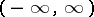with the weight function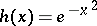. The standardized Hermite polynomials are defined by the Rodrigues formula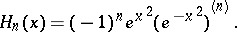The most commonly used formulas are: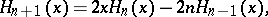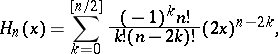The first few Hermite polynomials are: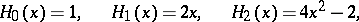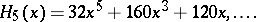The polynomial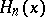satisfies the differential equation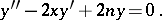The orthonormal Hermite polynomials are defined byThe Hermite polynomials with leading coefficient one have the formFourier series in Hermite polynomials in the interior ofbehave analogous to trigonometric Fourier series.

In mathematical statistics and probability theory one uses the Hermite polynomials corresponding to the weight function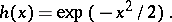The definition of Hermite polynomials is encountered in P. Laplace . A detailed study of them was published by P.L. Chebyshev in 1859 (see ). Later, these polynomials were studied by Ch. Hermite . V.A. Steklov  proved that the set of them is dense in the space of square-summable functions with the weight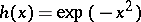on the whole real line.

How to Cite This Entry:
Hermite polynomials. Encyclopedia of Mathematics. URL: http://encyclopediaofmath.org/index.php?title=Hermite_polynomials&oldid=14720
This article was adapted from an original article by P.K. Suetin (originator), which appeared in Encyclopedia of Mathematics - ISBN 1402006098. See original article